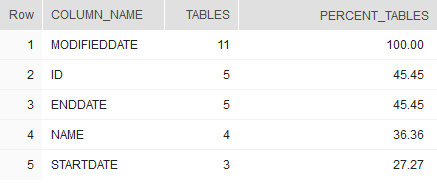## Find most popular column names in Snowflake

Article for:

This query lets you find out most popular column names.

## Query

``````select c.column_name as column_name,
count(*) as tables,
cast(100.0 * count(*) /
(select count(*) from information_schema.tables
where table_type = 'BASE TABLE')
as decimal(10,2))
as percent_tables
from information_schema.tables t
inner join information_schema.columns c on
c.table_schema = t.table_schema and c.table_name = t.table_name
where t.table_type = 'BASE TABLE'
group by c.column_name
having count(*) > 1
order by count(*) desc;
``````

## Columns

• column_name - column name
• tables - number of tables that have particular column name
• percent_tables - percentage of tables with column with that name

## Rows

• One row represents particular column name (each name appears only once)
• Scope of rows: all colum names that appear at least twice (in two tables)
• Ordered by the most popular first

## Sample results

As you can see MODIFIEDDATE column exists in 11 tables, which is all of them (100%).0